## How to Limit Data Entry in a Cell in Excel

This post will guide you how to limit cell entries to specific length and number of digits in Excel. How do I limit data entry to allow only text or only numbers entered into in a cell using Data Validation option in Excel. How to limit entries to numeric values in Excel.

Assuming that you want to limit data entry for a range of cells in your worksheet, such as: only allow to enter text values or numeric values in the selected range of cells. How to do it. You can refer to the following introduction.

## Limit Data Entry to Allow Only Text

If you want to only allow entering Text values in the selected range of cells, you can use the Data Validation feature to achieve it. Just do the following steps:

#1 select the range of cells that you want to limit data entry.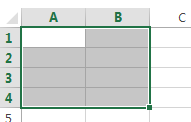#2 go to DATA tab, click Data Validation command under Data Tools group. And the Data Validation dialog will open.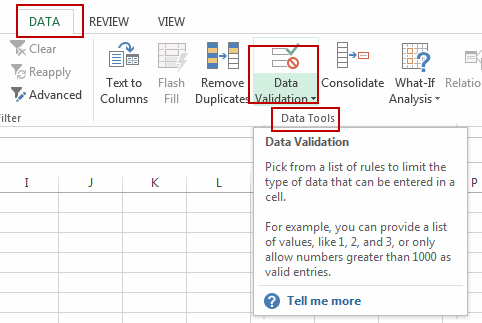#3 click Settings tab, and select Custom from the Allow drop down list box. And then type the following formula based on the ISTEXT function in Formula Text box. And click Ok button.

=ISTEXT(A1)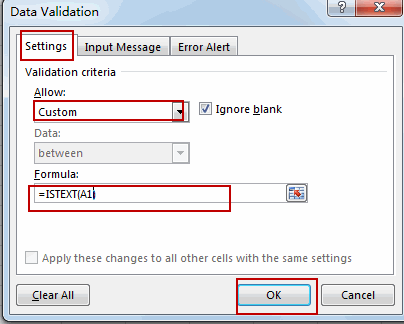This formula will check if the cell value is a text value or not, if TRUE, it returns TRUE, otherwise, returns FALSE, and the Data Validation rules will be triggered.

Note: A1 is the first cell in your selected range.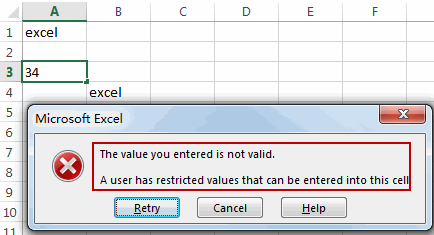## Limit Data Entry to Allow Only Numeric Values

If you want to only allow entering numeric values in the selected range of cells, you can use the Data validation feature in combination with the ISNUMBER function to achieve the result. Do the following steps:

#1 select the range of cells that you want to limit data entry.#2 go to DATA tab, click Data Validation command under Data Tools group. And the Data Validation dialog will open.#3 click Settings tab, and select Custom from the Allow drop down list box. And then type the following formula based on the ISNUMBER function in Formula Text box. And click Ok button.

=ISNUMBER(A1)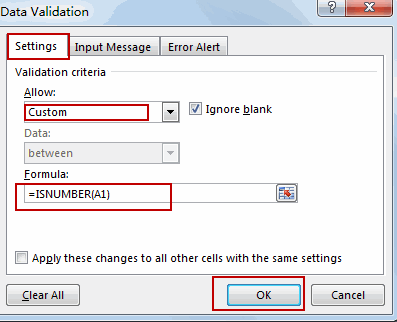The ISNUMBER function returns TRUE when cell value is a number and returns FALSE if not.

Note: A1 is the first cell in your selected range.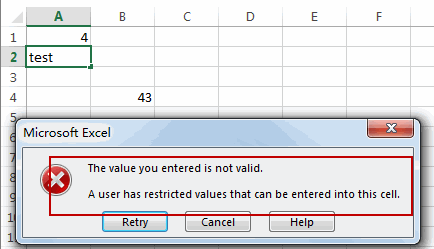## Limit Maximum Cell Value

If you want to limit data entries are not greater than or euqal to a given value (200) in a range, just do the following steps:

#1 Select the range of cells that you want to limit maximum cell value.#2 go to DATA tab, click Data Validation command under Data Tools group. And the Data Validation dialog will open.#3 click Settings tab in the opening Data Validation dialog, select Whole number in the allow drop down list box. And select less or equal to from the Data drop down list box. And then enter a numeric value 200 into text box of Maximum. Click OK button.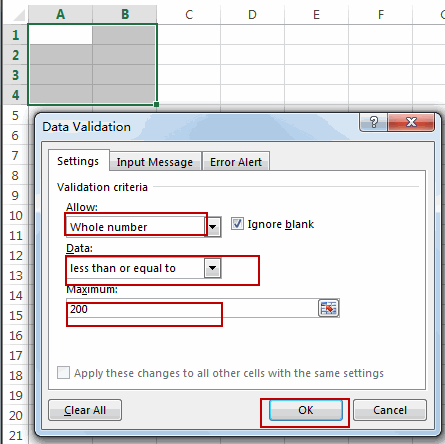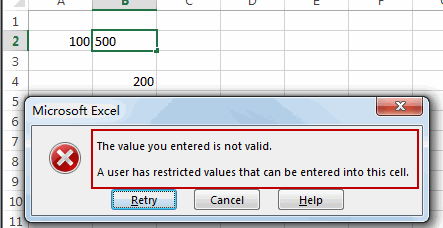Video: Limit Data Entry

### Related Functions

• Excel ISNUMBER function
The Excel ISNUMBER function returns TRUE if the value in a cell is a numeric value, otherwise it will return FALSE.The syntax of the ISNUMBER function is as below:= ISNUMBER (value)…
• Excel ISTEXT function
The Excel ISTEXT function used to check if a value is text. If so, returns TRUE; if the text is not text, the function will return FALSE.The syntax of the ISTEXT function is as below:=ISTEXT(value)…

## Excel ISTEXT Function

This post will guide you how to use Excel ISTEXT function with syntax and examples in Microsoft excel.

### Description

The Excel ISTEXT function used to check if a value is text. If so, returns TRUE; if the text is not text, the function will return FALSE. So you can use the ISTEXT function to check for a value that is text in Excel.

The ISTEXT function is a build-in function in Microsoft Excel and it is categorized as an Information Function.

The ISTEXT function is available in Excel 2016, Excel 2013, Excel 2010, Excel 2007, Excel 2003, Excel XP, Excel 2000, Excel 2011 for Mac.

### Syntax

The syntax of the ISTEXT function is as below:

`=ISTEXT(value)`

Where the ISTEXT function argument is:

• Value -This is a required argument. The value that you want to test.

### Excel ISTEXT Function Example

The below examples will show you how to use Excel ISTEXT Function to check if a value is text. And the ISTEXT function will return TRUE when value is a text string.

#1 checks whether the value in Cell B1 is a text string, using the following formula:

`= ISTEXT (B1)`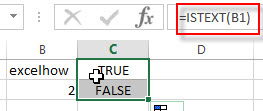### Related Functions

• Excel ISNUMBER Function
The Excel ISNUMBER function returns TRUE if the value in a cell is a numeric value, otherwise it will return FALSE.The syntax of the ISNUMBER function is as below:=ISNUMBER (value)
• Excel ISERR Function
The Excel ISERR function returns TRUE if the value is any error value except #N/A.The syntax of the ISERR function is as below:= ISERR (value)…
• Excel ISError Function
The Excel ISERROR function used to check for any error type that excel generates and it returns TRUE for any error type, such as: #N/A, #VALUE!,#REF!,#DIV0!, #NAME?, etc. The syntax of the ISERROR function is as below:= ISERROR (value)…
• Excel ISBlank Function
The Excel ISBLANK function returns TRUE if the value is blank or null.The syntax of the ISBLANK function is as below:=ISBLANK (value)…
• Excel ISNA Function
The Excel ISNA function used to check if a cell contains the #N/A error, if so, returns TRUE; otherwise, the ISNA function returns FALSE. The syntax of the ISNA function is as below:=ISNA(value)…
• Excel ISNONTEXT Function
The Excel ISNONTEXT function used to check if a value is text. If so, returns FALSE; if the text is not text, the function will return TRUE. The syntax of the ISNONTEXT function is as below:=ISNONTEXT (value)…
• Excel ISREF Function
The Excel ISREF function used to check if a value is a valid reference. If so, returns TRUE; if the value is not a reference, the function will return FALSE. The syntax of the ISREF function is as below:=ISREF(value)…